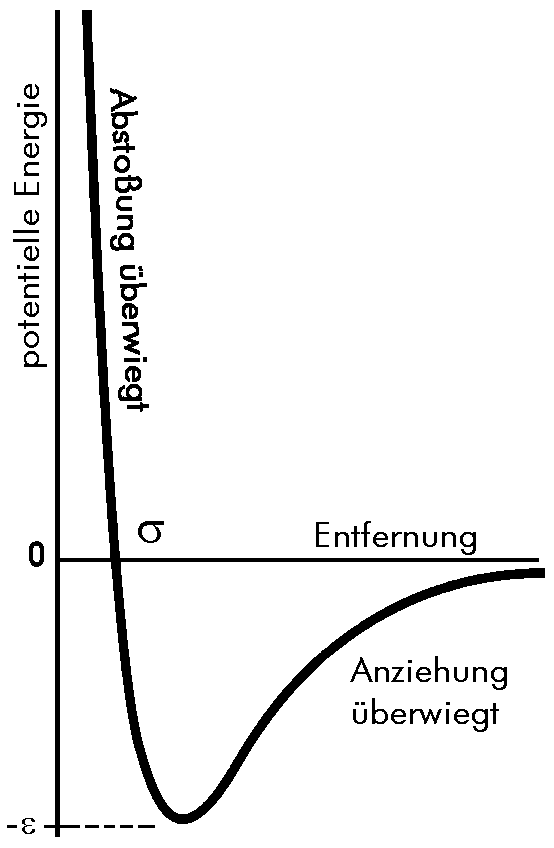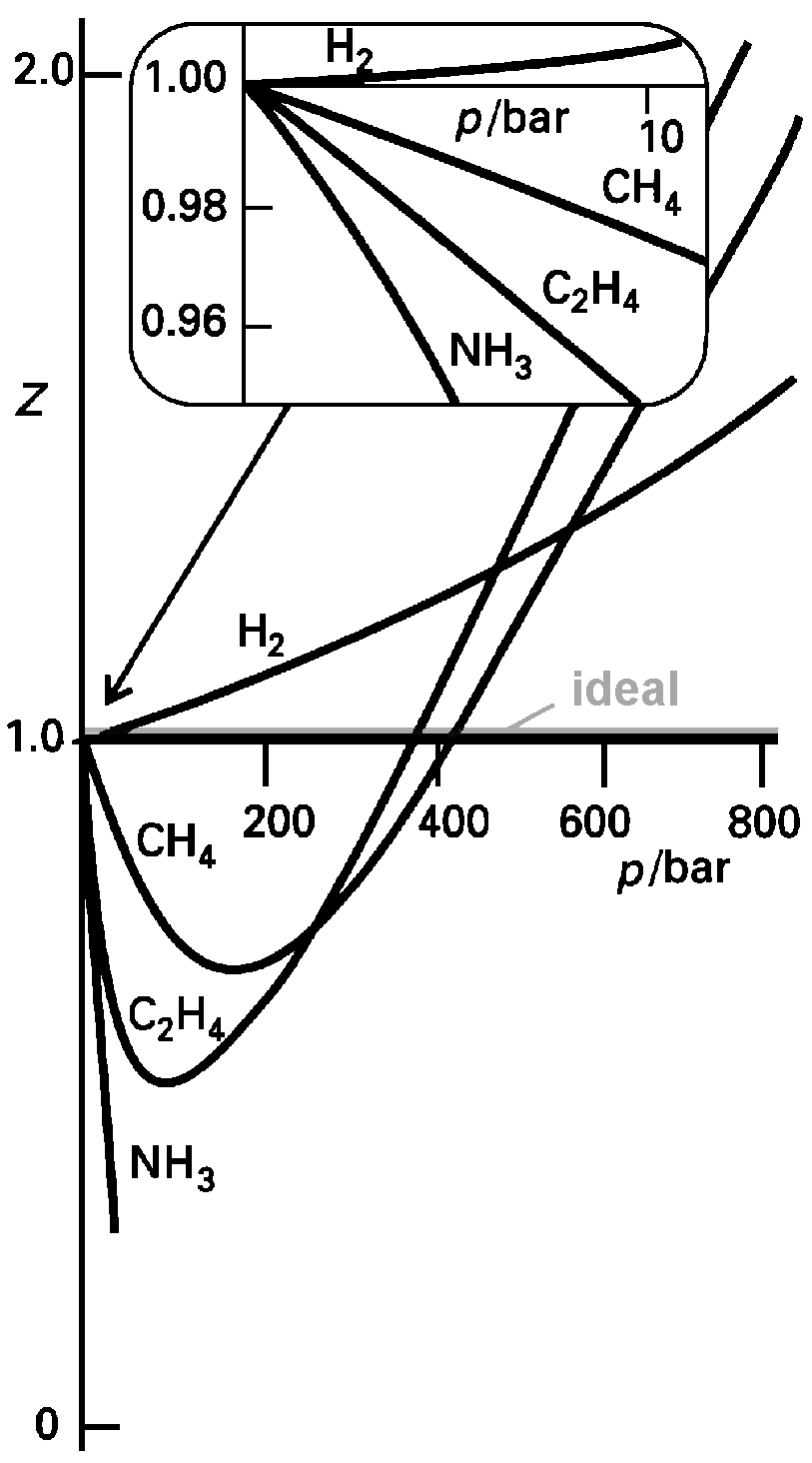# Ideal behavior should be

Real gasesFig. 1: The change in the potential energy of two molecules as a function of the distance (Lennard-Jones 6-12 potential). Large positive energies at small distances come about because the repulsive interactions predominate here. At a medium distance, the forces of attraction dominate and the potential energy is clearly negative. When the distance between the molecules becomes sufficiently large, the two no longer interact with each other; the potential energy becomes zero.

Real gases do not exactly fulfill the equation of state of the ideal gas. The higher the pressure and the lower the temperature, the more significant the deviations are; they have their most pronounced effect at the point of condensation to the liquid. The reason for the deviation of real gases from ideal behavior is the intermolecular interaction: mutual repulsion of the molecules favors expansion, forces of attraction favor compression. The repulsion between neutral molecules is a force with a short range (also compared to the molecule diameter), which only becomes significant when the molecules are almost touching each other (see figure). Such small mean intermolecular distances are only to be expected with a high particle density. It is mainly caused by the electrostatic repulsion between the outer electron clouds of the molecules. The following applies to the power of this repulsion: FR. ~ - r-13. On the other hand, forces of attraction have a relatively large range, they act across a few molecular diameters. The forces of attraction are due to a special arrangement of electrons in one molecule relative to another molecule. Hence, they are of interest at intervals of medium length; if there is a large intermolecular distance (at the outer right edge of the figure) they no longer play a role. The forces of attraction, also called London or dispersion forces, are approximately inversely proportional to the 7th power of the distance r, FA. ~ r-7. In general it is easier to consider the potential energy V (r) instead of the force, F = - dV (r) / dr. For the potential, as the sum of repulsion and attraction, one then obtains

 V (r) = 4 ε [ (σ/r)12 - (σ/r)6 ]

where ε is the maximum attraction energy (depth of potential). For r = σ and r → ∞ we get V (r) = 0. This potential is called Lennard-Jones 6-12 potential.
Regardless of the specific shape of the interaction potential, a gas behaves ideally when the molecules are far apart so that intermolecular interactions have no influence - that is, at low pressure. At moderate pressure, attraction dominates because the distance between two molecules is only a few particle diameters. Such a gas should be more compressible than an ideal one. With a further increase in pressure, the repulsive forces ultimately predominate, so that the gas is difficult to compress.

The compression factorFig. 2: The variation of the compression factorZ = pVm / RT with the pressure for some gases at 0 ° C. For an ideal gas, Z = 1 applies at any pressure. The curves approach the value 1 for p → 0 with different slopes.
The dependence of the intermolecular forces on the distance between the particles can be determined with the help of the compression factor Z

illustrate. For an ideal gas one always gets Z = 1; the deviation from 1 is therefore a measure of the deviation from ideal behavior. Z is applied as a function of the pressure. The figure shows some experimental values ​​for Z. At very low pressures, the gases behave almost ideally; Z ≈ 1; at very high pressures, Z is always greater than 1, i.e. the gases are more difficult to compress than an ideal gas (pVm> RT). Particle repulsion dominates. At moderate pressure, Z <1 for most gases, i.e. the attractive forces are decisive and the gas is comparatively easy to compress.The data protection declaration of the TU Braunschweig applies to this website with the exception of Sections VI, VII and VIII.